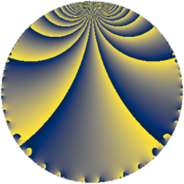Properties

 Label 6160.2.a.xLevel $6160$ Weight $2$ Character orbit 6160.a Self dual yes Analytic conductor $49.188$ Analytic rank $0$ Dimension $2$ CM no Inner twists $1$

Related objects

Newspace parameters

 Level: $$N$$ $$=$$ $$6160 = 2^{4} \cdot 5 \cdot 7 \cdot 11$$ Weight: $$k$$ $$=$$ $$2$$ Character orbit: $$[\chi]$$ $$=$$ 6160.a (trivial)

Newform invariants

 Self dual: yes Analytic conductor: $$49.1878476451$$ Analytic rank: $$0$$ Dimension: $$2$$ Coefficient field: $$\Q(\sqrt{2})$$ Defining polynomial: $$x^{2} - 2$$ Coefficient ring: $$\Z[a_1, a_2, a_3]$$ Coefficient ring index: $$2$$ Twist minimal: no (minimal twist has level 3080) Fricke sign: $$-1$$ Sato-Tate group: $\mathrm{SU}(2)$

$q$-expansion

Coefficients of the $$q$$-expansion are expressed in terms of $$\beta = 2\sqrt{2}$$. We also show the integral $$q$$-expansion of the trace form.

 $$f(q)$$ $$=$$ $$q + \beta q^{3} - q^{5} - q^{7} + 5 q^{9} +O(q^{10})$$ $$q + \beta q^{3} - q^{5} - q^{7} + 5 q^{9} + q^{11} + ( 2 - \beta ) q^{13} -\beta q^{15} + ( 2 + \beta ) q^{17} -\beta q^{21} + 4 q^{23} + q^{25} + 2 \beta q^{27} -2 q^{29} + ( -4 + \beta ) q^{31} + \beta q^{33} + q^{35} + ( 6 - 2 \beta ) q^{37} + ( -8 + 2 \beta ) q^{39} + ( 6 - \beta ) q^{41} + ( 4 + 2 \beta ) q^{43} -5 q^{45} -\beta q^{47} + q^{49} + ( 8 + 2 \beta ) q^{51} + ( -2 - 2 \beta ) q^{53} - q^{55} + ( 4 - \beta ) q^{59} + ( 6 + \beta ) q^{61} -5 q^{63} + ( -2 + \beta ) q^{65} + 2 \beta q^{67} + 4 \beta q^{69} + 2 \beta q^{71} + ( -6 - \beta ) q^{73} + \beta q^{75} - q^{77} + q^{81} + 2 \beta q^{83} + ( -2 - \beta ) q^{85} -2 \beta q^{87} + ( -6 + 4 \beta ) q^{89} + ( -2 + \beta ) q^{91} + ( 8 - 4 \beta ) q^{93} + ( 2 + 4 \beta ) q^{97} + 5 q^{99} +O(q^{100})$$ $$\operatorname{Tr}(f)(q)$$ $$=$$ $$2 q - 2 q^{5} - 2 q^{7} + 10 q^{9} + O(q^{10})$$ $$2 q - 2 q^{5} - 2 q^{7} + 10 q^{9} + 2 q^{11} + 4 q^{13} + 4 q^{17} + 8 q^{23} + 2 q^{25} - 4 q^{29} - 8 q^{31} + 2 q^{35} + 12 q^{37} - 16 q^{39} + 12 q^{41} + 8 q^{43} - 10 q^{45} + 2 q^{49} + 16 q^{51} - 4 q^{53} - 2 q^{55} + 8 q^{59} + 12 q^{61} - 10 q^{63} - 4 q^{65} - 12 q^{73} - 2 q^{77} + 2 q^{81} - 4 q^{85} - 12 q^{89} - 4 q^{91} + 16 q^{93} + 4 q^{97} + 10 q^{99} + O(q^{100})$$

Embeddings

For each embedding $$\iota_m$$ of the coefficient field, the values $$\iota_m(a_n)$$ are shown below.

For more information on an embedded modular form you can click on its label.

Label $$\iota_m(\nu)$$ $$a_{2}$$ $$a_{3}$$ $$a_{4}$$ $$a_{5}$$ $$a_{6}$$ $$a_{7}$$ $$a_{8}$$ $$a_{9}$$ $$a_{10}$$
1.1
 −1.41421 1.41421
0 −2.82843 0 −1.00000 0 −1.00000 0 5.00000 0
1.2 0 2.82843 0 −1.00000 0 −1.00000 0 5.00000 0
 $$n$$: e.g. 2-40 or 990-1000 Significant digits: Format: Complex embeddings Normalized embeddings Satake parameters Satake angles

Atkin-Lehner signs

$$p$$ Sign
$$2$$ $$1$$
$$5$$ $$1$$
$$7$$ $$1$$
$$11$$ $$-1$$

Inner twists

This newform does not admit any (nontrivial) inner twists.

Twists

By twisting character orbit
Char Parity Ord Mult Type Twist Min Dim
1.a even 1 1 trivial 6160.2.a.x 2
4.b odd 2 1 3080.2.a.g 2

By twisted newform orbit
Twist Min Dim Char Parity Ord Mult Type
3080.2.a.g 2 4.b odd 2 1
6160.2.a.x 2 1.a even 1 1 trivial

Hecke kernels

This newform subspace can be constructed as the intersection of the kernels of the following linear operators acting on $$S_{2}^{\mathrm{new}}(\Gamma_0(6160))$$:

 $$T_{3}^{2} - 8$$ $$T_{13}^{2} - 4 T_{13} - 4$$ $$T_{17}^{2} - 4 T_{17} - 4$$ $$T_{19}$$ $$T_{23} - 4$$

Hecke characteristic polynomials

$p$ $F_p(T)$
$2$ $$T^{2}$$
$3$ $$-8 + T^{2}$$
$5$ $$( 1 + T )^{2}$$
$7$ $$( 1 + T )^{2}$$
$11$ $$( -1 + T )^{2}$$
$13$ $$-4 - 4 T + T^{2}$$
$17$ $$-4 - 4 T + T^{2}$$
$19$ $$T^{2}$$
$23$ $$( -4 + T )^{2}$$
$29$ $$( 2 + T )^{2}$$
$31$ $$8 + 8 T + T^{2}$$
$37$ $$4 - 12 T + T^{2}$$
$41$ $$28 - 12 T + T^{2}$$
$43$ $$-16 - 8 T + T^{2}$$
$47$ $$-8 + T^{2}$$
$53$ $$-28 + 4 T + T^{2}$$
$59$ $$8 - 8 T + T^{2}$$
$61$ $$28 - 12 T + T^{2}$$
$67$ $$-32 + T^{2}$$
$71$ $$-32 + T^{2}$$
$73$ $$28 + 12 T + T^{2}$$
$79$ $$T^{2}$$
$83$ $$-32 + T^{2}$$
$89$ $$-92 + 12 T + T^{2}$$
$97$ $$-124 - 4 T + T^{2}$$